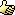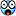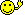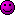CodeGuru Home VC++ / MFC / C++ .NET / C# Visual Basic VB Forums Developer.com

# Thread: The haystack is growing...

1. ## Re: The haystack is growing...

But... For me, the really interesting question regarding this sequence (and this somehow brings it close to the MU-puzzle in Gödel, Escher Bach...) is: Will this sequence always produce growing strings - or is there a chance that they get shorter along the way?What I mean is: It's pretty obvious that a row in this sequence can be shorter than its predecessor - for example, the successor of "1111111" will be "71", or the successor of "222333" will be "3233"...But: Will this ever happen with the sequence starting with the seed "1"? And if not: Why not?Reply With Quote

2. ## Re: The haystack is growing...Originally Posted by gstercken
Nice sequence...The next one is "1113213211", right?
Good cracking !Reply With Quote

3. ## Re: The haystack is growing...Originally Posted by gstercken
Nice sequence...The next one is "1113213211", right?
Well, I knew you know the answer.Let's see if anybody can find the next one.

Will this ever happen with the sequence starting with the seed "1"? And if not: Why not?
Allow me to ask a simpler question: will a string ever encounter a 4?Reply With Quote

4. ## Re: The haystack is growing...

Next...
31131211131221Ever contain a 4
I do not think so (given the starting seed). Without giving away the algorythm, just one key word "Consecutive"Reply With Quote

5. ## Re: The haystack is growing...

Yes, you got it right.

I checked until the string with length 234241786. No 4 in it.Reply With Quote

6. ## Re: The haystack is growing...Originally Posted by cilu
Yes, you got it right.

I checked until the string with length 234241786. No 4 in it.But perhaps with a length of 234241787???Reply With Quote

7. ## Re: The haystack is growing...

13211311123113112211

I dont think it'll ever have a 4 .... It's just not in..Reply With Quote

8. ## Re: The haystack is growing...

Another interesting question is can an element in the sequence be collapsed to that it is an compacted representation of the same value?Reply With Quote

9. ## Re: The haystack is growing...Originally Posted by TheCPUWizard
Another interesting question is can an element in the sequence be collapsed to that it is an compacted representation of the same value?
I dont know..

Considering the sequence, wont it break when you try to compact any part of it ???Reply With Quote

10. ## Re: The haystack is growing...Originally Posted by TheCPUWizard
Another interesting question is can an element in the sequence be collapsed to that it is an compacted representation of the same value?
Not sure I'm following... Can you give an example?Reply With Quote

11. ## Re: The haystack is growing...Originally Posted by cilu
I checked until the string with length 234241786. No 4 in it.Interesting...Now I wonder what a proof for that would look like?<thinking>

// Same for the steadily growing strings, btw... Did your empirical approach support that too, Marius?Reply With Quote

12. ## Re: The haystack is growing...Originally Posted by gstercken
Interesting...Now I wonder what a proof for that would look like?<thinking>

// Same for the steadily growing strings, btw... Did your empirical approach support that too, Marius?Just solve the following for "t":

Code:
```D(t+1) = (sigma(K=1,LOG(D(t)*10)-LOG(D(t)*10)%1,((D(t)-D(t)%10^(LOG(D(t))-
LOG(D(t))%1)+sigma(S=1,LOG(D(t))-LOG(D(t))%1,(((sigma(R=1,LOG(D(t)*10)
-LOG(D(t)*10)%1-1,ABS((D(t)*10-D(t)*10%10^(R+1))%10^(R+2)/10-(D(t)*10-D(t)*
10%10^R)%10^(R+1)))/10)-(sigma(R=1,LOG(D(t)*10)-LOG(D(t)*10)%1-1,ABS((D(t)*
10-D(t)*10%10^(R+1))%10^(R+2)/10-(D(t)*10-D(t)*10%10^R)%10^(R+1)))/10)
%10^(S-1))%10^S+1)%(((sigma(R=1,LOG(D(t)*10)-LOG(D(t)*10)%1-1,ABS((D(t)
*10-D(t)*10%10^(R+1))%10^(R+2)/10-(D(t)*10-D(t)*10%10^R)%10^(R+1)))/10)-(
sigma(R=1,LOG(D(t)*10)-LOG(D(t)*10)%1-1,ABS((D(t)*10-D(t)*10%10^(R+1))%10
^(R+2)/10-(D(t)*10-D(t)*10%10^R)%10^(R+1)))/10)%10^(S-1))%10^S+.5)*2*(D(t)
-D(t)%10^(S-1))%10^S))-(D(t)-D(t)%10^(LOG(D(t))-LOG(D(t))%1)+sigma(S=1,
LOG(D(t))-LOG(D(t))%1,(((sigma(R=1,LOG(D(t)*10)-LOG(D(t)*10)%1-1,ABS((D(t)
*10-D(t)*10%10^(R+1))%10^(R+2)/10-(D(t)*10-D(t)*10%10^R)%10^(R+1)))/10)
-(sigma(R=1,LOG(D(t)*10)-LOG(D(t)*10)%1-1,ABS((D(t)*10-D(t)*10%10^(R+1))%
10^(R+2)/10-(D(t)*10-D(t)*10%10^R)%10^(R+1)))/10)%10^(S-1))%10^S+1)%(((
sigma(R=1,LOG(D(t)*10)-LOG(D(t)*10)%1-1,ABS((D(t)*10-D(t)*10%10^(R+1))%
10^(R+2)/10-(D(t)*10-D(t)*10%10^R)%10^(R+1)))/10)-(sigma(R=1,LOG(D(t)*
10)-LOG(D(t)*10)%1-1,ABS((D(t)*10-D(t)*10%10^(R+1))%10^(R+2)/10-(D(t)*
10-D(t)*10%10^R)%10^(R+1)))/10)%10^(S-1))%10^S+.5)*2*(D(t)-D(t)%10^(S
-1))%10^S))%10^(K-1))%10^K/10^(K-1)*100^(2*sigma(N=1,K,(((D(t)-D(t)%
10^(LOG(D(t))-LOG(D(t))%1)+sigma(S=1,LOG(D(t))-LOG(D(t))%1,(((sigma(R=1,
LOG(D(t)*10)-LOG(D(t)*10)%1-1,ABS((D(t)*10-D(t)*10%10^(R+1))%10^(R+2)/10-
(D(t)*10-D(t)*10%10^R)%10^(R+1)))/10)-(sigma(R=1,LOG(D(t)*10)-LOG(D(t)*
10)%1-1,ABS((D(t)*10-D(t)*10%10^(R+1))%10^(R+2)/10-(D(t)*10-D(t)*10%10^R)%
10^(R+1)))/10)%10^(S-1))%10^S+1)%(((sigma(R=1,LOG(D(t)*10)-LOG(D(t)*10)%
1-1,ABS((D(t)*10-D(t)*10%10^(R+1))%10^(R+2)/10-(D(t)*10-D(t)*10%10^R)%
10^(R+1)))/10)-(sigma(R=1,LOG(D(t)*10)-LOG(D(t)*10)%1-1,ABS((D(t)*10-D(t)
*10%10^(R+1))%10^(R+2)/10-(D(t)*10-D(t)*10%10^R)%10^(R+1)))/10)%10^(S-1))
%10^S+.5)*2*(D(t)-D(t)%10^(S-1))%10^S))-(D(t)-D(t)%10^(LOG(D(t))-LOG(D(t))
%1)+sigma(S=1,LOG(D(t))-LOG(D(t))%1,(((sigma(R=1,LOG(D(t)*10)-LOG(D(t)*10)
%1-1,ABS((D(t)*10-D(t)*10%10^(R+1))%10^(R+2)/10-(D(t)*10-D(t)*10%10^R)%10^
(R+1)))/10)-(sigma(R=1,LOG(D(t)*10)-LOG(D(t)*10)%1-1,ABS((D(t)*10-D(t)*10%
10^(R+1))%10^(R+2)/10-(D(t)*10-D(t)*10%10^R)%10^(R+1)))/10)%10^(S-1))%10^
S+1)%(((sigma(R=1,LOG(D(t)*10)-LOG(D(t)*10)%1-1,ABS((D(t)*10-D(t)*10%10^
(R+1))%10^(R+2)/10-(D(t)*10-D(t)*10%10^R)%10^(R+1)))/10)-(sigma(R=1,
LOG(D(t)*10)-LOG(D(t)*10)%1-1,ABS((D(t)*10-D(t)*10%10^(R+1))%10^(R+2)/
10-(D(t)*10-D(t)*10%10^R)%10^(R+1)))/10)%10^(S-1))%10^S+.5)*2*(D(t)-D(t)%
10^(S-1))%10^S))%10^(N-1))%10^N+1)%(((D(t)-D(t)%10^(LOG(D(t))-LOG(D(t))%
1)+sigma(S=1,LOG(D(t))-LOG(D(t))%1,(((sigma(R=1,LOG(D(t)*10)-LOG(D(t)*
10)%1-1,ABS((D(t)*10-D(t)*10%10^(R+1))%10^(R+2)/10-(D(t)*10-D(t)*10%10^
R)%10^(R+1)))/10)-(sigma(R=1,LOG(D(t)*10)-LOG(D(t)*10)%1-1,ABS((D(t)*10-
D(t)*10%10^(R+1))%10^(R+2)/10-(D(t)*10-D(t)*10%10^R)%10^(R+1)))/10)%10^
(S-1))%10^S+1)%(((sigma(R=1,LOG(D(t)*10)-LOG(D(t)*10)%1-1,ABS((D(t)*10-
D(t)*10%10^(R+1))%10^(R+2)/10-(D(t)*10-D(t)*10%10^R)%10^(R+1)))/10)-
(sigma(R=1,LOG(D(t)*10)-LOG(D(t)*10)%1-1,ABS((D(t)*10-D(t)*10%10^(R+1))%
10^(R+2)/10-(D(t)*10-D(t)*10%10^R)%10^(R+1)))/10)%10^(S-1))%10^S+.5)*2*
(D(t)-D(t)%10^(S-1))%10^S))-(D(t)-D(t)%10^(LOG(D(t))-LOG(D(t))%1)+
sigma(S=1,LOG(D(t))-LOG(D(t))%1,(((sigma(R=1,LOG(D(t)*10)-LOG(D(t)*10
)%1-1,ABS((D(t)*10-D(t)*10%10^(R+1))%10^(R+2)/10-(D(t)*10-D(t)*10%10^
R)%10^(R+1)))/10)-(sigma(R=1,LOG(D(t)*10)-LOG(D(t)*10)%1-1,ABS((D(t)*
10-D(t)*10%10^(R+1))%10^(R+2)/10-(D(t)*10-D(t)*10%10^R)%10^(R+1)))/
10)%10^(S-1))%10^S+1)%(((sigma(R=1,LOG(D(t)*10)-LOG(D(t)*10)%1-1,
ABS((D(t)*10-D(t)*10%10^(R+1))%10^(R+2)/10-(D(t)*10-D(t)*10%10^R)%
10^(R+1)))/10)-(sigma(R=1,LOG(D(t)*10)-LOG(D(t)*10)%1-1,ABS((D(t)*
10-D(t)*10%10^(R+1))%10^(R+2)/10-(D(t)*10-D(t)*10%10^R)%10^(R+1)))
/10)%10^(S-1))%10^S+.5)*2*(D(t)-D(t)%10^(S-1))%10^S))%10^(N-1))%10^
N+.5))))/100)+(sigma(K=1,LOG(D(t)*10)-LOG(D(t)*10)%1,100^(1+sigma(N=
1,K-1,2*((((sigma(R=1,LOG(D(t)*10)-LOG(D(t)*10)%1-1,ABS((D(t)*10-D(t)*
10%10^(R+1))%10^(R+2)/10-(D(t)*10-D(t)*10%10^R)%10^(R+1)))/10)-(
sigma(R=1,LOG(D(t)*10)-LOG(D(t)*10)%1-1,ABS((D(t)*10-D(t)*10%10^(R+1))%
10^(R+2)/10-(D(t)*10-D(t)*10%10^R)%10^(R+1)))/10)%10^(N-1))%10^N/10^(N-
1)+1)%(((sigma(R=1,LOG(D(t)*10)-LOG(D(t)*10)%1-1,ABS((D(t)*10-D(t)*10%
10^(R+1))%10^(R+2)/10-(D(t)*10-D(t)*10%10^R)%10^(R+1)))/10)-(sigma(R=
1,LOG(D(t)*10)-LOG(D(t)*10)%1-1,ABS((D(t)*10-D(t)*10%10^(R+1))%10^(R+
2)/10-(D(t)*10-D(t)*10%10^R)%10^(R+1)))/10)%10^(N-1))%10^N/10^(N-1)+
.5)))))/10)```
(Yes, that is really the algorythm!!!)Reply With Quote

13. ## Re: The haystack is growing...

I made this C++ code to display the first n elements of the series.

Code:
```#include <string>
#include <vector>
#include <fstream>
#define LIMIT 20
using namespace std;
string parse(string str1);
string eval(vector<int> vec);

int main(int argc, char* argv[])
{
int i=1;
string str1="1";
ofstream logData;
logData.open("CG.txt",ios::out);
for(i=1;i<LIMIT;i++) {
printf("%s\n",str1.c_str());
logData << str1.c_str() << "\n";
str1 = parse(str1);
}
logData.close();
return 0;
}

string parse(string str1) {
int len = str1.length();
int j;
vector<int> numList;
for(int i=0;i<len;i++) {
j = str1[i] - '0';
numList.push_back(j);
}
return eval(numList);
}

string eval(vector<int> vec) {
int len = vec.size();
int cnt1=1;
vector<int> vec_new;
for(int i=1;i<=len;i++) {
if(vec[i-1]==vec[i]) {
cnt1++;
}
if(vec[i-1]!=vec[i]) {
vec_new.push_back(cnt1);
vec_new.push_back(vec[i-1]);
cnt1=1;
}
}
string str;
for(i=0;i<vec_new.size();i++) {
str+=vec_new[i]+'0';
}
return str;
}```
enjoyReply With Quote

14. ## Re: The haystack is growing...

SweetAlthough I would NOT have put the call to eval inside of parse. This is because parse now does not return a "parsed" string, it returns an "evaluated" one (which is at odds with the function name.....

[picky, picky, picky, picky, picky, picky, picky]Reply With Quote

15. ## Re: The haystack is growing...Originally Posted by TheCPUWizard
SweetAlthough I would NOT have put the call to eval inside of parse. This is because parse now does not return a "parsed" string, it returns an "evaluated" one (which is at odds with the function name.....

[picky, picky, picky, picky, picky, picky, picky]Picky indeedAnd 4 really doesn't come out in the sequence (through brute force evaluation)Reply With Quote

####Posting Permissions

• You may not post new threads
• You may not post replies
• You may not post attachments
• You may not edit your posts
•